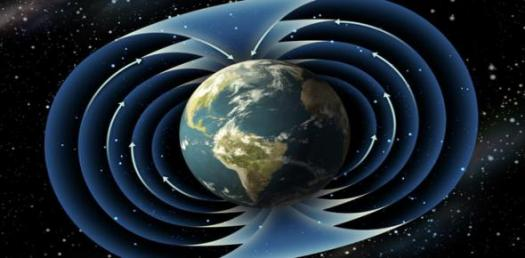# Earth's Magnetic Fields Quiz For 6th Grade

10 Questions | Total Attempts: 144SettingsThe earth's magnetic field refers to the force that extends from the internal part of the earth into the outer space. The strength of the geomagnetic field is as a result of the intense electric current from the earth's core. Are you in the know? Take this quiz if you do.

Related Topics
• 1.
What is the process by which escapes convection currents from the earth's core called?
• A.

Microteslas

• B.

Geodynamo

• C.

Paleomagnetism

• D.

Magnetosphere

• 2.
What part of the earth is under the influence of the magnetic field ?
• A.

Biosphere

• B.

Lithosphere

• C.

Magnetosphere

• D.

Hydrosphere

• 3.
What is the study of past magnetic field of the Earth called?
• A.

Paleomagnetism

• B.

Quantal magnetism

• C.

Magnetostratigraphy

• D.

Spiral magnetism

• 4.
How is the intensity of the earth's magnetic field measured?
• A.

Gauss

• B.

Tesla

• C.

Newton

• D.

Ampere

• 5.
Which of these is positive for an eastward deviation of the field relative to true north?
• A.

Magnetic declination

• B.

Geodynamo

• C.

Celestial pole

• D.

Geographical declination

• 6.
Which of these is most likely to repel?
• A.

Like poles

• B.

North poles

• C.

Unlike poles

• D.

South poles

• 7.
Which of these is most likely to attract?
• A.

North poles

• B.

South poles

• C.

Unlike poles

• D.

Like poles

• 8.
Where is the point where the magnetic field is vertical?
• A.

Local pole

• B.

South pole

• C.

Global pole

• D.

North pole

• 9.
Which of these is not part of the magnetosphere?
• A.

Bow shock

• B.

Magnetosheath

• C.

Northern tail

• D.

Eastern tail lobe

• 10.
What continent houses the northern, magnetic pole?
• A.

Africa

• B.

Asia

• C.

Oceania

• D.

Australia